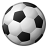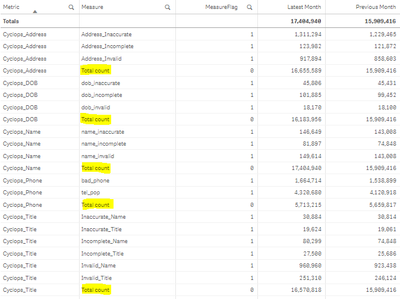Announcements
You can succeed best and quickest by helping others to succeed. Join the conversation.
cancel
Showing results for
Did you mean:Creator III

## Expression Help Required

Hi all,

Just a quick question from me, I'm struggling to get the correct expression to give me a quality score. The data is laid out in the following way:

 RunDate Metric Measure Value 01/03/2023 Cyclops_Title Total count 15909416 01/03/2023 Cyclops_Title Invalid_Name 923438 01/03/2023 Cyclops_Title Inaccurate_Name 30814 01/03/2023 Cyclops_Title Incomplete_Name 74848 01/03/2023 Cyclops_Title Invalid_Title 246124 01/03/2023 Cyclops_Title Inaccurate_Title 19061 01/03/2023 Cyclops_Title Incomplete_Title 25686

So I'd need a % score in a new column where it divides the measure by the Total count...can anyone help me out please?

Labels (1)
• ### General Question

2 Solutions

Accepted SolutionsMVP

@samvile18  It all depends on how you want to calculate your share. I am assuming for each Date and Metrics you would want share, but if date do not take part just use Metrics in Total qualifier

``````Sum({<Measure-={'Total count'}>} Value)/Sum(TOTAL <RunDate,Metrics>{<Measure={'Total count'}>} Value)

or

Sum({<Measure-={'Total count'}>} Value)/Sum(TOTAL <Metrics>{<Measure={'Total count'}>} Value)``````Partner - Creator II

Sum({<Measure-={'Total count'}>} Value)/Sum(TOTAL <Metric> {<Measure={'Total count'}>} Value)9 RepliesPartner - Creator II

Maybe try this :

Sum({<Measure-={'Total count'}>} Value) / Sum({<Measure={'Total count'}>} Value)

And then you need to format the measure in %Creator III
Author

That's what I tried originally but I can't get it to return anything other than null...no idea why it isn't working.MVP

@samvile18  providing sample with expected output will be useful to get correct answers quickly.Creator III
AuthorI've copied the table I have in Qlik and I've added the data coming in too. Essentially I'd be hoping to have a % Score column next to each measure. So each measure under Cyclops_Address would use the relevant total.Partner - Creator II

Sum({<Measure-={'Total count'}>} Value)/Sum(TOTAL {<Measure={'Total count'}>} Value)Creator III
Author

How would you extend this to cater for the different groups of Metric? Like in the following picture:So anything under Cyclops_Address would need to use the Cyclops_Address Total count...whereas Cylcops_DOB would need to use the Cyclops_DOB Total count...and so on.MVP

@samvile18  It all depends on how you want to calculate your share. I am assuming for each Date and Metrics you would want share, but if date do not take part just use Metrics in Total qualifier

``````Sum({<Measure-={'Total count'}>} Value)/Sum(TOTAL <RunDate,Metrics>{<Measure={'Total count'}>} Value)

or

Sum({<Measure-={'Total count'}>} Value)/Sum(TOTAL <Metrics>{<Measure={'Total count'}>} Value)``````Partner - Creator II

Sum({<Measure-={'Total count'}>} Value)/Sum(TOTAL <Metric> {<Measure={'Total count'}>} Value)Creator III
Author

You two are absolute legends - I thought Total but I was putting it in the wrong place because I'm an idiot. Thanks very muchTags
Community Browser